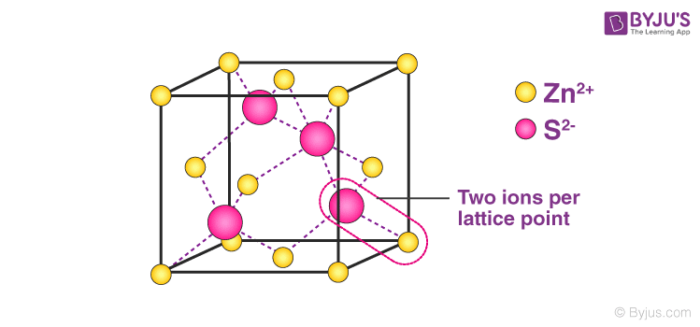Checkout JEE MAINS 2022 Question Paper Analysis : Checkout JEE MAINS 2022 Question Paper Analysis :

# Density of Unit Cell - Primitive unit cell

## Definition of Unit Cell

The smallest group of atoms which has the overall symmetry of a crystal, and from which the entire lattice can be built up by repetition in three dimensions is termed as Unit Cell. Crystalline solids exhibit a regular and repeating pattern of constituent particles.

The diagrammatic representation of the three-dimensional arrangement of constituent particles in a crystal, in which each particle is depicted as a point in space is known as crystal lattice.## What is a Lattice?

A lattice is a framework, resembling a three-dimensional, periodic array of points, on which a crystal is built. In 1850, M. A. Bravais showed that identical points can be arranged spatially to produce 14 types of regular pattern. These 14 space lattices are known as Bravais lattices.

The crystal lattice of a solid can be described in terms of its unit cell. A crystal lattice is made up of a very large number of unit cells where every lattice point is occupied by one constituent particle. The unit cell can be seen as a three-dimensional structure containing one or more atoms.

We can determine the volume of this unit cell with the knowledge of the dimensions of the unit cell. For example: if we have a unit cell of an edge “a”, the volume of the unit cell can be given as “a3”. Density of a unit cell is given as the ratio of mass and volume of unit cell. The mass of a unit cell is equal to the product of the number of atoms in a unit cell and the mass of each atom in a unit cell.

Mass of unit cell = number of atoms in unit cell × mass of each atom = z × m

Where, z = number of atoms in unit cell,

m = Mass of each atom

Mass of an atom can be given with the help of Avogadro number and molar mass as:

$$\begin{array}{l} \frac { M}{N_A} \end{array}$$

Where, M = molar mass

Volume of unit cell, V = a3

=> Density of unit cell =

$$\begin{array}{l} \frac {mass~ of~ unit~ cell}{volume~ of~ unit ~cell}\end{array}$$

=> Density of unit cell =

$$\begin{array}{l} \frac {m}{V} \end{array}$$
=
$$\begin{array}{l} \frac {z~×~m}{a^3}\end{array}$$
=
$$\begin{array}{l} \frac {z~×~M}{a^3~×~N_A} \end{array}$$

Thus, with the knowledge of number of atoms in a unit cell, edge length and molar mass we can determine the density of a unit cell.

## A general expression for density of unit cell for various cases has been derived below:

1. Primitive unit cell: In a primitive unit cell, the number of atoms in a unit cell, z is equal to one. Hence, density is given as:

Density of unit cell =

$$\begin{array}{l} \frac {1~×~M }{a^3~×~N_A} \end{array}$$

2. Body-centered cubic unit cell: In body-centered cubic unit cell, the number of atoms in a unit cell, z is equal to two. Hence, density is given as:

Density of unit cell =

$$\begin{array}{l} \frac {2~×~M }{a^3~×~N_A} \end{array}$$

3. Face-centered cubic unit cell: In face-centered cubic unit cell, the number of atoms in a unit cell, z is equal to four. Hence, density of unit cell is given as:

Density of unit cell =

$$\begin{array}{l} \frac {4~×~M }{a^3~×~N_A} \end{array}$$

## Frequently Asked Questions – FAQs

### What is the volume of a unit cell?

The unit cell volume (V) is equal to the cubed cell-edge length (a). In a face-centered cubic structure, there would be four atoms per unit cell and the nickel density in this structure would be four times as high.

### What is the formula of density of any crystal?

We measure up the mass of one unit cell to the mass of all the atoms present in that same cell. The number of atoms is going to depend on the type of cell it is. So we multiply the number of atoms “n” into the mass of each atom “m” to achieve the mass of a unit cell.

### What is difference between primitive cell and unit cell?

Explain the distinction between a unit cell and a primal cell. Only at the corners of a cell do primitive cells allow lattice points. At edges, face centres and body centres, unit cell facilitates lattice points. By investigating a unit cell, we can examine the crystal as a whole.

### What are the types of primitive unit cell?

There are seven primitive systems of crystals; cubic, tetragonal, orthorhombic, hexagonal, monoclinic, rhomboedral and triclinic. The structure of their crystallographic axes and angles differs between them.

### What is unit cell in solid state?

The most fundamental and lowest volume-consuming repeated form of any solid is a unit cell. It is used to visually simplify the solids in which crystalline patterns organise themselves. The network is called a lattice as the unit cell repeats itself.

For detailed discussion on calculation of density of unit cell, download BYJU’S – The Learning App.

Test your Knowledge on Density of unit cell!

#### 1 Comment

1. Syed Imran

Its a very amezing for study, i hope my future safe in this